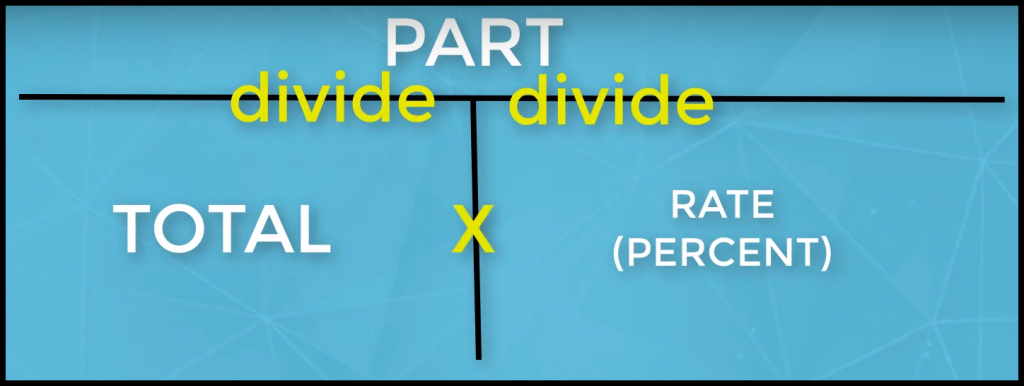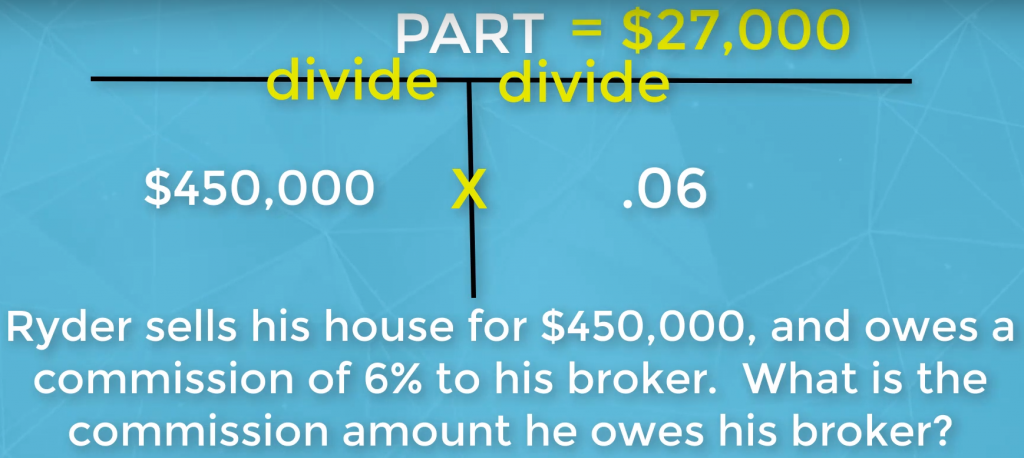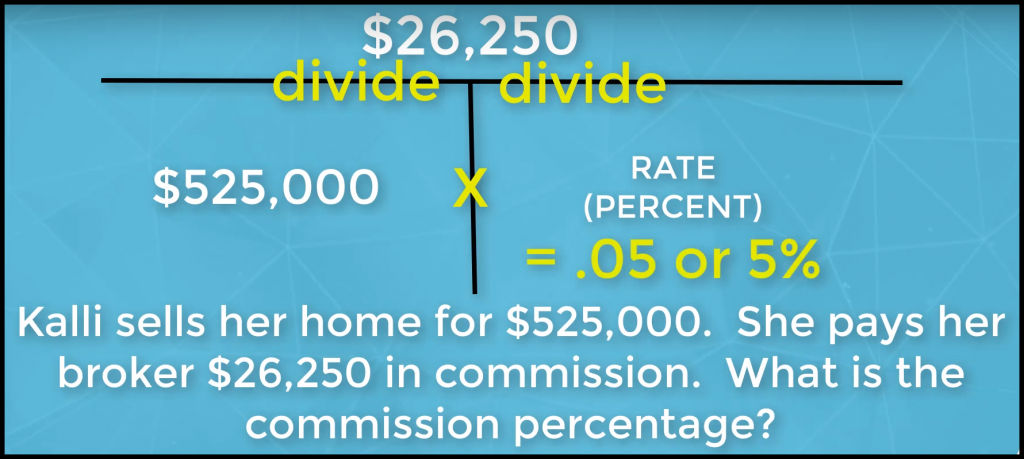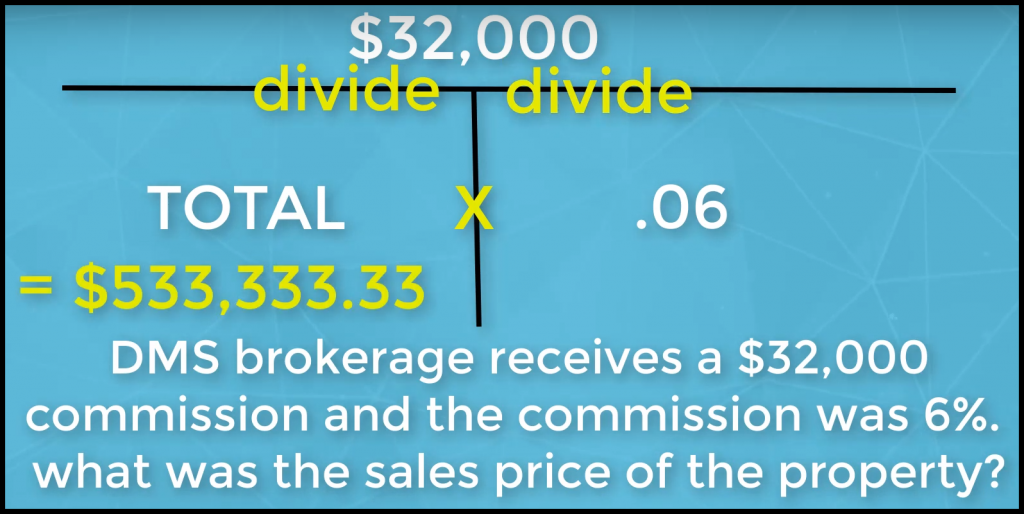# Real Estate Exam Math Made Easy

We have an incredible solution that will make real estate exam math easy for you.  Our top secret “t” chart will help you find the answer for up to 75% of the math questions on the real estate exam.  It doesn’t matter whether you are a math expert or not.  This chart is so simple you will wonder why no one told you about it before.  Straight from our course, the video below fully explains this top secret chart.  Additionally, it is fully described in the text to follow.

## Why is real estate math so hard?

Real estate exam math is so hard because there are so many formulas to remember.  Our “t” chart will make it real estate math easy for you decipher.  If you encounter a math question on the exam that includes two variables and you are asked to solve for the third you can use this chart.  Knowing what to do when presented with a math question will also ease your testing anxiety.  You can learn more about managing testing anxiety here.

## Do you want to learn a massive secret to real estate math?  Here it is:Above you will find the secret to mastering real estate math.   It would help if you wrote this on your scratch paper when you take your state exam.  Then you can refer to it whenever you encounter a math question.  The best thing about this chart is, it will NEVER change.  The question data will change, but the chart will not.

To be more specific, you will see in the chart above; there are three spots you can input information.  If you are given the total, you will input it on the bottom left of the chart.  On the bottom left of the chart, you can input the total, if that is what you are given.  If presented a percentage, you will always input it into the bottom right of the chart.  The top of the chart is for the part of a total.

If you have the part and one of the pieces of information on the bottom, you will divide the part by the number on the bottom to solve for the answer.  If you are provided both the total and rate, then you will multiply.  So, you will either divide or multiply.  Simple as that.  You will have two numbers, and you will either divide or multiply to find the third.

You are now a real estate exam math wizard!

## Is there any way I can get tripped up using this “t” chart?

Yes, there is.  Here is a real estate exam math tip you must know.  When using a percentage, you always move the decimal over two spaces to the left.  In other words, any math you are going to do, a percentage would be input by using a decimal and then inputting the rate.  For example, if provided a commission rate of 5% in a question, you would input it as .05 when computing an answer for the test.  Additionally, if you come up with a solution for a rate, and your calculator says .03, that would be 3%.

Now you have one less thing to get confused about math on your real estate exam.

## How do you correctly use this incredible real estate math tool?

Great question.  We want you to master real estate exam math.  Below is an example of a math question you may encounter on your real estate exam:As you see in the above question, they provide two pieces of information.  You need to plug it into the chart.  The \$450,000 is the total and goes in the bottom left.  The 6% is the rate and goes in the bottom right as .06.  You multiply them to come up with the answer of \$27,000, which is the part.  Simple as that.

## Let’s check out another sample question that will help you master real estate exam math.

Check this out:As you can see, again you are provided with two pieces of data.  In the chart, you place the total in the bottom left.  You input the part in the top.  When you go top to bottom, you divide.  \$26,250 divided by \$525,000 gives you .05, or 5%.  Again, simple as that.

Are you ready for one more?  You want all the real estate math tips you can get your hands on, right?

## Check out our last sample math question:You plug the percentage into the bottom right.  We also have the part of the total, which goes on the top.  When we go top to bottom, we divide.  \$32,000 divided by .06 is \$533,333.33.  There you have it – yet another real estate math question you easily solved.  Your real estate math knowledge has now increased tremendously.

By the way, if you want to ace your exam, our course includes over 100 practice real estate math questions.  Each one of them consists of a detailed written explanation which will walk you through computing the answer.

Our course also includes over 2 1/2 hours of video instruction covering 150 testable concepts.

## What else can help me prepare to pass my real estate licensing exam on my first attempt?

Other tips to help you pass your real estate licensing exam on your first attempt:

Real Estate Test Taking Tips

How to Pass the Real Estate Exam

Also, check out our question of the day videos on our YouTube channel:

PassMasters Real Estate Exam Prep YouTube Channel